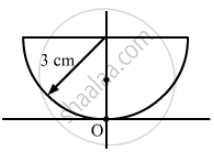Advertisement Remove all ads

# Solve the Previous Problem If the Paperweight is Inverted at Its Place So that the Spherical Surface Touches the Paper. - Physics

Sum

Solve the previous problem if the paperweight is inverted at its place so that the spherical surface touches the paper.

Advertisement Remove all ads

#### Solution

Given,
Taking the radius of the paperweight as its thickness = 3 cm
Refractive index of the paperweight (μg) = 3/2
Refractive index of the air (μ1) = 1Image shift is given by:

$∆ t = \left( 1 - \frac{1}{\mu} \right)t$

$= \left( 1 - \frac{2}{3} \right)3$

$= \left( \frac{1}{3} \right)3$

$= 1 \text{ cm }$
The upper surface of the paperweight is flat and the spherical spherical surface is in contact with the printed letter.
Therefore, we will take it as a simple refraction problem.

Hence, the image will appear 1 cm above point A.

Is there an error in this question or solution?
Advertisement Remove all ads

#### APPEARS IN

HC Verma Class 11, 12 Concepts of Physics 1
Chapter 18 Geometrical Optics
Q 45 | Page 415
Advertisement Remove all ads
Advertisement Remove all ads
Share
Notifications

View all notifications

Forgot password?